# Venn diagram 2 & 3 Example#

Here shows how to draw venn diagram

```import numpy as np
import pandas as pd

import milkviz as mv
```

## Data input for venn diagram#

There are three types of data input

1. Specific a list of sets

2. Specific a list of list, intersections between lists will be computed for you, duplicates will considred

3. A list of number denotes the regions in venn diagram in the following order: venn2 used (10, 01, 11) or venn3 used (100, 010, 110, 001, 101, 011, 111)

```np.random.seed(0)
list_sets = [set([1,2,3,4,5,13,100]),
set([4,5,6,7,8,100,101]),
set([4,5,10,11,12,13,101])]
list_list = [np.random.randint(0, 20, 10),
np.random.randint(0, 20, 30),
np.random.randint(0, 20, 15)]
list_area = (1, 1, 1, 2, 1, 2, 2)
```

## Create venn2#

```mv.venn([set([1,2,3,4]), set([4,5,6,7])])
```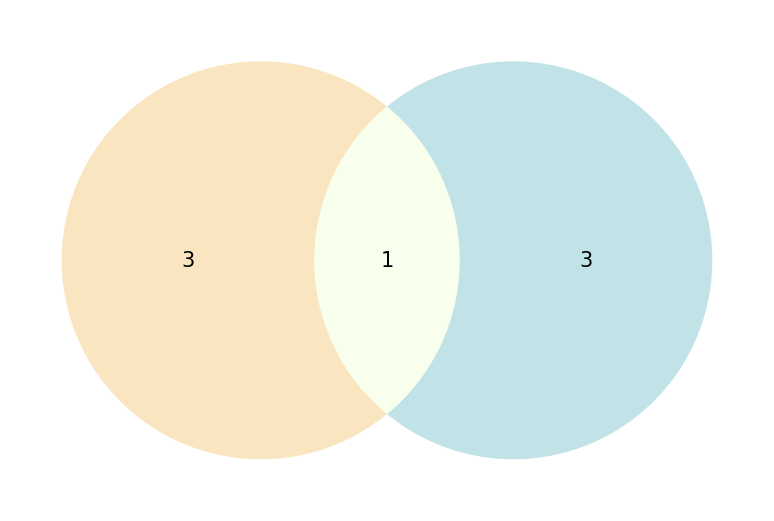```<AxesSubplot: >
```

## Create the venn with set#

```mv.venn(list_sets, names=['A', 'B', 'C'])
```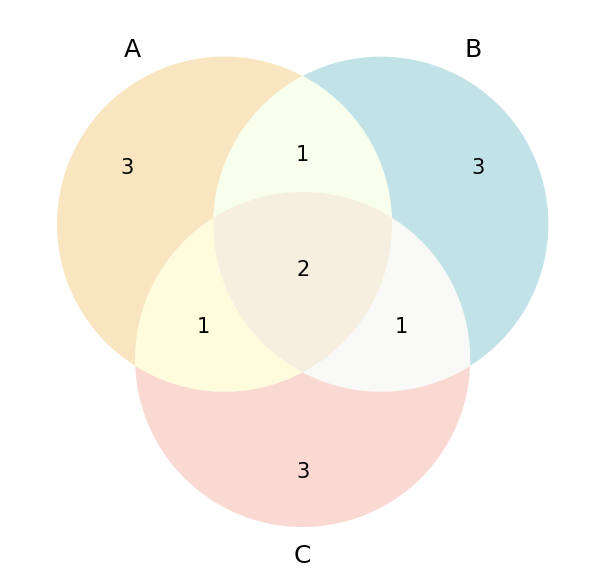```<AxesSubplot: >
```

## Create the venn with list#

```mv.venn(list_list)
```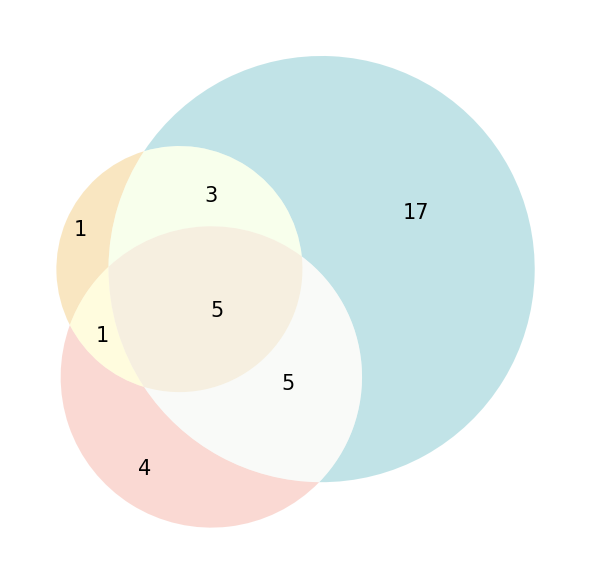```<AxesSubplot: >
```

## Create the venn with area#

```mv.venn(list_area)
```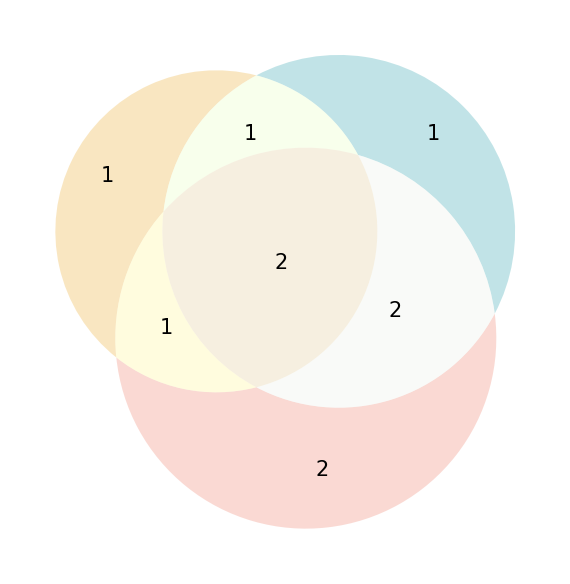```<AxesSubplot: >
```

## Create unweighted venn#

```mv.venn(list_list, weighted=False)
```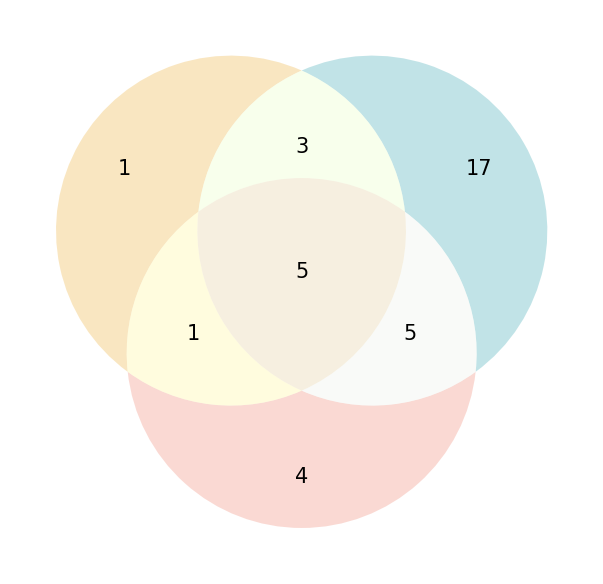```<AxesSubplot: >
```

Total running time of the script: ( 0 minutes 1.871 seconds)

Gallery generated by Sphinx-Gallery